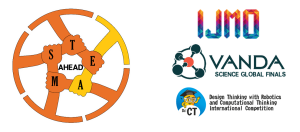# SYLLABUS

## SINGA SYLLABUS

1. Number upto 1000
2. Addition and Subtraction within 1000
3. Multiplication and Division of 2 3 4 5 & 10
4. length
5. Mass
6. Volume
7. Money
8. Fractions
9. Time
10. Picture Graph 12D & 3D Shapes
11. Heuristics

### For Grade 3 & 4:

1. Number Upto 100000
2. Factors And Multiples
3. 4 Operations of Whole Numbers within 100000
4. Fractions
5. Decimals
6. Time
7. Area And Perimeters
8. Angles
9. Properties of Rectangle and Squares.
10. Line Symmetry
11. Table, Bar graphs and Line graphs
12. Money
13. Length, Mass and Volume
14. Parallel and Perpendicular lines
15. Heuristics

### For Grade 5 & 6:

1. Numbers upto 10 Millions
2. 4 Operation of wholesome number within 10 millions
3. 4 operations of fractions
4. 4 operations of decimals
5. Ratio
6. Percentage
7. Area and Perimeter of composite Figures
8. Angles
9. Properties of Triangle and Quadrilaterals
1. Volume of Cube and Cuboid
2. Rate and Speed
3. Average
4. Algebra
5. Pie Graph
6. Nets Solid Figures
7. Heuristics

### For Grade 7 & 8:

1. Numbers upto 10 Millions
2. 4 Operation of wholesome number within 10 millions
3. 4 operations of fractions
4. 4 operations of decimals
5. Ratio
6. Percentage
7. Area and Perimeter of composite Figures
8. Angles
9. Properties of Triangle and Quadrilaterals
1. Volume of Cube and Cuboid
2. Rate and Speed
3. Average
4. Algebra
5. Pie Graph
6. Nets Solid Figures
7. Heuristics

### For Grade 9, 10 , 11 & 12:

1. Numbers and Number Patterns
2. Angles and Polygons
3. Mensuration, Arc Length and Area of Sector
4. Equations, Functions and Polynomial
5. Inequalities
6. Indices and Surds
7. Coordinate Geometry and and Circles
8. Pythagoras theorem, Further Trigonometry and Application Of Trigonometry
9. Trigonometric Functions, identities and Equations
1. Congurence and Similarity, Area and Volume of Similar Figures and Solids
2. Geometry and Properties of  Circles
3. Set Language and Notations
4. Probability
5. Statistical Data Analysis
6. Vector in two dimensions
7. Binomial theorem
8. Matrices
Scroll to TopGlobal Final (Indonesia) Registration date has Extended

Last date is 10th October 2023